Published: 09 October 2015

# Simulation of rolling bearing vibration in diagnostics

Robert Kostek1
Bogdan Landowski2
Łukasz Muślewski3
1, 2, 3University of Science and Technology, Bydgoszcz, Poland
Corresponding Author:
Robert Kostek
Views 147

#### Abstract

This article presents results on simulation of deep groove ball bearings vibrations. Contacts between rolling elements and the races can be modelled as the Hertzian contact. This contact is on-linear, thus introduces non-linear spring forces, in consequence this vibrations are non-linear. Positions of rolling elements are functions of time, thus these vibrations can be considered as parametric vibrations. In consequence rolling bearings are active non-linear elements, which excite vibrations. This approach reflects the nature of rolling bearings vibrations. The following phenomena have been observed: bistability, jump of amplitude, opposing period-doubling bifurcations “a bubble”, period-doubling bifurcations leading to chaos, bifurcation directly leading to chaos, chaotic vibrations, windows of periodic vibrations, “noisy periodicity”, and chaotic explosion. Moreover, amplitudes of vibrations have been presented against clearance. This provides an opportunity to select failure modes. Unfortunately, relations clearance-amplitude and amplitude-clearance are ambiguous, which makes machinery diagnostic a difficult issue.

## 1. Introduction

Rolling bearings are key elements in machines, and influence their reliability, thus their vibrations are an important issue [1-14]. Rolling bearings vibrations are parametric, because positions of rolling elements are the function of time, in spite of certain slip. These vibrations are nonlinear, because the Hertzian contact is nonlinear. And thus, characteristic phenomena are observed: bistability, jump of amplitude, bifurcation directly leading to chaos, chaotic vibrations, and windows of periodic vibrations. These phenomena make the relations of clearance – amplitude and amplitude – clearance ambiguous. If, bistability or chaotic vibrations are observed, then various magnitudes of amplitudes can be observed for the same clearance. It should be mentioned, that chaotic vibrations are nonperiodic, which leads to non-recurrent results. Whereas, bistability, bifurcation directly leading to chaos, and windows of periodic vibrations lead to jumps of amplitude. In consequence changes of clearance, which are below 0.5 m, can lead to large changes of amplitude – jumps of amplitude. The aforementioned phenomena are simulated with numerical methods, because analytical methods are very difficult in this case [5, 6, 15]. Summarising, the contact introduces strong non-linearity even to very simple systems . Most of articles present fragmentary studies on ball bearings vibrations, they are focused on one phenomenon, thus there is a need to present study for a wide range of clearance.

## 2. Model of rolling bearing

The deep groove ball bearing 608Z is modelled as a mechanical system (Fig. 1). First, the housing is modelled as a fixed rigid body. Next, the shaft is modelled as a rigid body, which has a mass and three degree of freedom. The shaft rotates, and vibrates in two directions – $x$ and $y$. Then, the balls and the Hertzian contacts are modelled as non-linear massless springs. Finally, the shaft and the inner race rotate in counter clockwise direction, thus the balls circulate in the counter clockwise direction. In consequence, stiffness of bearing is a function of time which excite vibrations.

The following forces act on the shaft: spring force, damping force, friction force, external force, and inertial force. The spring forces ${R}_{n}$ are described by the following equations:

1
${\delta }_{n}=d+0.5{D}_{1}-0.5{D}_{2}+{x}_{1}\mathrm{c}\mathrm{o}\mathrm{s}\alpha +{y}_{1}\mathrm{s}\mathrm{i}\mathrm{n}\alpha ,$
2
${\delta }_{n}>0,{R}_{n}=K{\delta }_{n}^{1.5}$${R}_{n}=0,$

where: ${\sigma }_{n}$ – is the sum of contact deflections which corresponds to $n$th ball, $d$ – represents the diameter of balls, ${D}_{1}$ – denotes the diameter of inner race, ${D}_{2}$ – is a diameter of the outer race, ${x}_{1}$, ${y}_{1}$ – are displacement of the shaft, $\alpha$ – denotes angular position of $n$th ball, ${R}_{n}$ – is the contact force acting on $n$th ball $N$, and $K$ – is the coefficient of contact stiffens N/m1.5. Components of damping force ${F}_{d}$ are described by the following equations:

3
${F}_{dx}=-c\stackrel{˙}{x},$
4
${F}_{dy}=-c\stackrel{˙}{y},$

where: ${F}_{dx}$ and ${F}_{dy}$ are $x$ and $y$ components of damping force $N$, $c$ – denotes the coefficient of damping $c=$ 200 (Ns)/m. This model of damping was used previously. The friction force of rolling contact is described by the following expression:

5
${F}_{otn}={R}_{n}\mu ,$

where: ${F}_{otn}$ – denotes rolling friction force acting on $n$th ball, and $\mu$ – is coefficient of rolling friction. The external force $F$ is constant during simulation, and is presented in Table 1. The equations of motion in two directions are presented below:

6
$\stackrel{¨}{x}={m}^{-1}\left({F}_{x}-\stackrel{˙}{x}c+\mathrm{\Sigma }{F}_{otxn}+\mathrm{\Sigma }{R}_{xn}\right),$
7
$\stackrel{¨}{y}={m}^{-1}\left({F}_{y}-\stackrel{˙}{y}c+\mathrm{\Sigma }{F}_{otyn}+\mathrm{\Sigma }{R}_{yn}\right),$

where: $m$ – represents equivalent mass attached to shaft.

Fig. 1a) The deep groove ball bearing 608Z and b) its model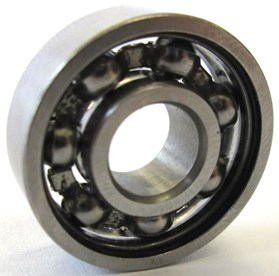a)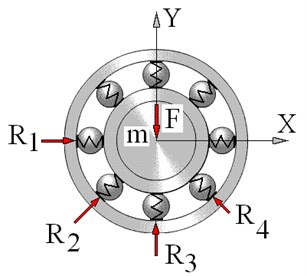b)

## 3. Results of simulation

Three amplitudes of vibration are depicted against clearance at Fig. 2. They are described by the following equations:

8
${A}_{xP-P}=\frac{{x}_{max}-{x}_{min}}{2},$
9
${A}_{xRMS}={\left(\frac{\mathrm{\Sigma }{\left({x}_{i}-{x}_{mean}\right)}^{2}}{j}\right)}^{0.5},$
10
${A}_{xAVG}=\frac{\mathrm{\Sigma }\left|{x}_{i}-{x}_{mean}\right|}{j},$

where: ${A}_{xP-P}$ – denotes peak-to-peak amplitude, ${x}_{max}$– is the largest magnitude, ${x}_{min}$– represents the smallest magnitude, ${A}_{xRMS}$ – is root mean square amplitude, ${x}_{mean}$ – denotes arithmetic mean of $x$, $j$ – is the number of samples, whereas ${A}_{xAA}$ – is the average amplitude. The arithmetic mean is included in two equations because, in practice, the place of the origin is unknown.

 Description Magnitudes Units Diameter of balls 3.9685 mm Number of balls 7 Inner race diameter 11.00 mm Inner race groove diameter 4.279 mm Outer race groove diameter 4.279 mm Young’s modulus 2.0e+5 MPa Poisson’s ratio 0.3 Coefficient of rolling friction 0.0015 Coefficient of damping 200 (Ns)/m $y$-component of the external force ${F}_{y}$ –331.5 N $x$-component of the external force ${F}_{x}$ 0 N Speed of the shaft 2953.9 rpm Mass of the inner race and shaft 1.854 kg

For small clearance (0-10 m) amplitudes are almost constant. Next the amplitudes generally rise, but local minima, jumps of amplitude, and bistability are observed. Then, for clearance being larger than 66 m, chaotic motion is observed. Chaotic motion at Fig. 2 is represented by a number of points, because various results are obtained for the same clearance. If a line is below these points, it means that a window of periodical vibrations is observed. Bifurcation diagram provides an opportunity to clearly present these phenomena.

Presented results (Fig. 2) show that the relations clearance – amplitude and amplitude – clearance are ambiguous. Nevertheless, amplitudes ${A}_{xRMS}$, ${A}_{xAVG}$, ${A}_{yRMS}$, ${A}_{yAVG}$ seem to be good state indicators, because of small number of local minima.

Bifurcation diagrams (Fig. 3) correspond to Fig. 2. First, periodical vibrations are observed (0-25.6 m), they are represented by a single line (Fig. 3(a)). Then, a bistability area is observed, thus jump of amplitude takes pace (Fig. 2(e) and 4(a)). In this case, booth periodical vibrations and chaotic vibrations can be obtained for the same clearance. Bifurcations directly leading to chaos, cascades of period-doubling bifurcations leading to chaos, “noisy periodicity” and windows of periodic motion are depicted in Fig. 4(a). Next, periodical vibrations are observed, which is two times larger than the period of excitation – double line (37.6-53 m) (Fig. 3(a), (b)). After bifurcation (53 m), the vibration period equals the period of excitation (single line). After that, bifurcation directly leading to chaos is observed for clearance being 66.6 m (Fig. 3(b) and 4(b)). This bifurcation significantly changes the amplitude, thus a jump of amplitude is observed at Fig. 2(e), (f). It should be mentioned, that within a large interval of chaotic motion, small windows of periodic vibrations are observed (66.6-100 m). A small window of periodic motion is magnified and depicted in Fig. 4(c), which shows: structure of this window, bifurcations and chaotic explosion. Lager windows of periodic motion are observed for clearance being near 78 m and 85 m (Fig. 3(b)). Some changes of amplitudes correspond to these windows of periodic motion (Fig. 2(e), (f)).Finally, second bistability is observed (Fig. 3(c) and 4(d)). Bifurcations directly leading to chaos, period-doubling cascade leading to chaos, “noisy periodicity”, jumps of amplitude, and opposing period-doubling bifurcations are observed. Both periodic and chaotic vibrations can be exited for this bistability. It should be mentioned that, a local minimum of amplitude corresponds to this bistability at Fig. 2. Finally, a large interval of chaotic motion with small windows of periodic motion is observed (Fig. 3(c), (d)). Summarising, bifurcation diagram is necessary to explain changes of amplitudes.

Fig. 2Amplitudes of vibrations against various magnitudes of clearance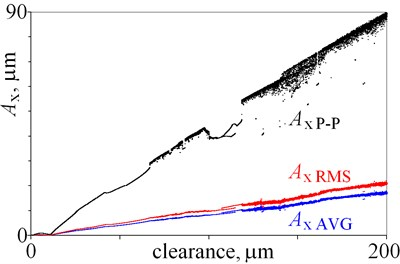a)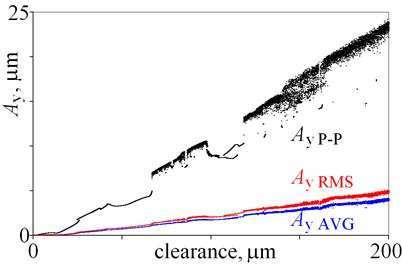b)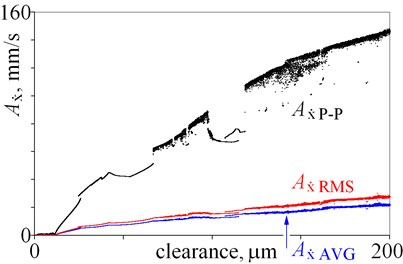c)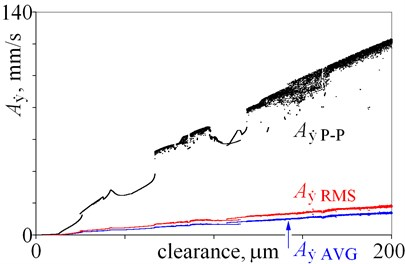d)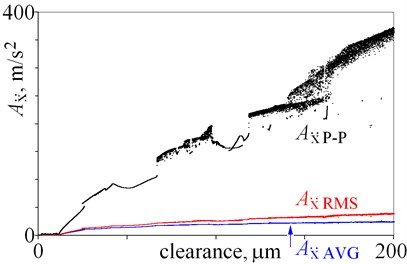e)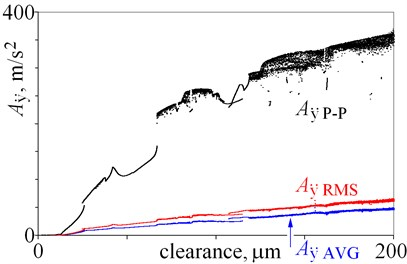f)

## 4. Conclusions

The relations of clearance and amplitude provide important information for machinery diagnostic, because failure modes (state indicators) can be identified. For the studied example, amplitudes of displacement seem to be the best. Moreover, before an expensive experimental study, results of simulation can be analysed. Local minima and jumps of amplitudes, which introduce difficulties into machinery diagnostic, can be explained on the basis of bifurcation diagrams. Local minima and jumps of amplitude make the relation of amplitude and clearance ambiguous. Finally, a number of nonlinear phenomena were observed for this model: bistability, jump of amplitude, opposing period-doubling bifurcations “a bubble”, period-doubling bifurcations leading to chaos, bifurcation directly leading to chaos, chaotic vibrations, windows of periodic vibrations, “noisy periodicity”, and chaotic explosion, which was not expected. This shows that linear models of rolling bearing can lead to large errors.

Fig. 3Bifurcation diagrams obtained for various magnitudes of clearance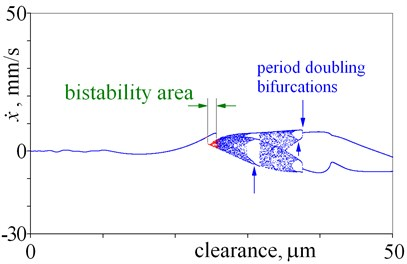a)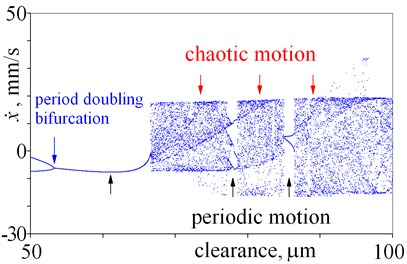b)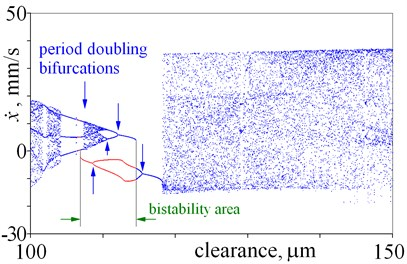c)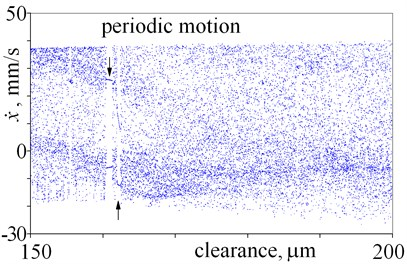d)

Fig. 4Details of bifurcation diagrams obtained for various magnitudes of clearance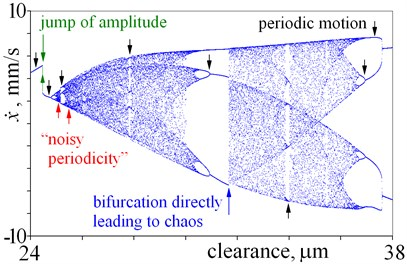a)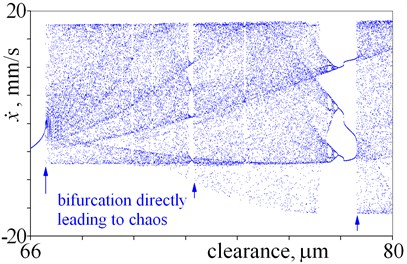b)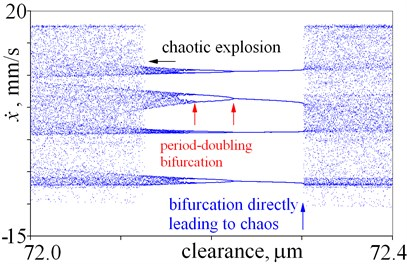c)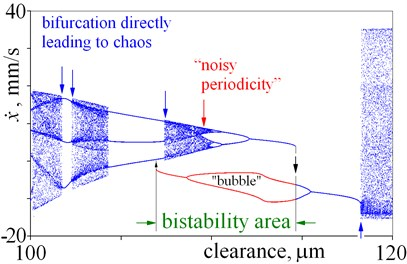d)